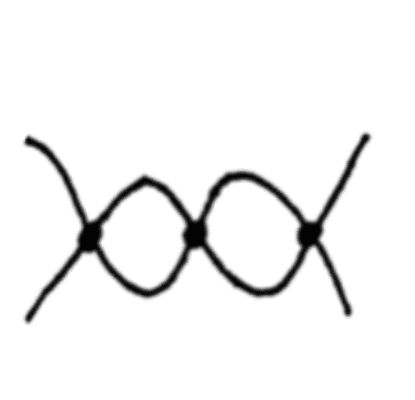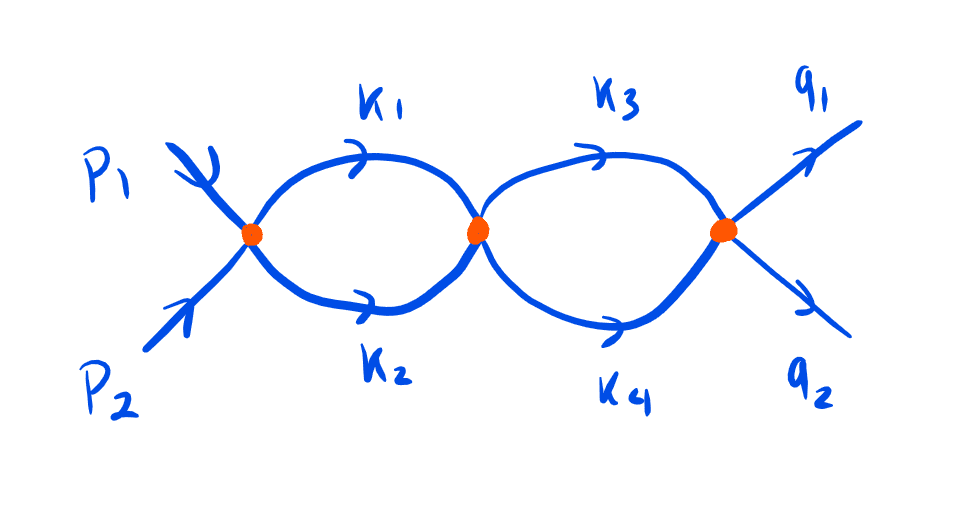# Amplitude of Feynman diagram in ##\phi^4## interactions

snypehype46
Homework Statement:
Calculate the amplitude of this Feynman diagram in momentum space for $\phi^4$ theory
Relevant Equations:
None
This is not really homework assigned to me but I wasn't sure where to post this.
I'm trying to work through the book "Quantum Field Theory for Gifted Amateurs" by Tom Lancaster. I'm doing the questions on Chapter 19 to understand how to draw Feynman diagrams and work out their amplitude. One of the exercises involved the computation of the amplitude of the following Feynman diagram in momentum space:What I got was the following:

$$2\pi ^4 \delta^{(4)} (p_1+p_2- q_1 -q_2) \frac{(-i \lambda)^3}{4} \int {\frac{d^4 k_1 d^4 k_2 d^4 k_3 d^4k_4}{(2\pi)^16}} \frac{i}{k_1^2 -m^2 +i\epsilon} \frac{i}{k_2^2 -m^2 +i\epsilon} \times \\ \frac{i}{(p_1 +p_2 - k_3)^2 -m^2 +i\epsilon} \frac{i}{(p_1 +p_2 - k_4)^2 -m^2 +i\epsilon}$$

This is the diagram I drew to compute thisBut it turns that the correct solution should be

$$2\pi ^4 \delta^{(4)} (p_1+p_2- q_1 -q_2) \frac{(-i \lambda)^3}{4} \int {\frac{d^4 k_1 d^4 k_2}{(2\pi)^8}} \frac{i}{k_1^2 -m^2 +i\epsilon} \frac{i}{k_2^2 -m^2 +i\epsilon} \times \\ \frac{i}{(p_1 +p_2 - k_1)^2 -m^2 +i\epsilon} \frac{i}{(p_1 +p_2 - k_2)^2 -m^2 +i\epsilon}$$

I don't quite get how to get rid of the ##k_3## and ##k_4## terms as they did in the solution. I assume this is due to the conservation of momentum but I'm not sure.

Last edited:

Quantour
Homework Statement:: Calculate the amplitude of this Feynman diagram in momentum space for $\phi^4$ theory
Relevant Equations:: None

This is not really homework assigned to me but I wasn't sure where to post this.
I'm trying to work through the book "Quantum Field Theory for Gifted Amateurs" by Tom Lancaster. I'm doing the questions on Chapter 19 to understand how to draw Feynman diagrams and work out their amplitude. One of the exercises involved the computation of the amplitude of the following Feynman diagram in momentum space:

View attachment 281695
What I got was the following:

$$2\pi ^4 \delta^{(4)} (p_1+p_2- q_1 -q_2) \frac{(-i \lambda)^3}{4} \int {\frac{d^4 k_1 d^4 k_2 d^4 k_3 d^4k_4}{(2\pi)^16}} \frac{i}{k_1^2 -m^2 +i\epsilon} \frac{i}{k_2^2 -m^2 +i\epsilon} \times \\ \frac{i}{(p_1 +p_2 - k_3)^2 -m^2 +i\epsilon} \frac{i}{(p_1 +p_2 - k_4)^2 -m^2 +i\epsilon}$$

This is the diagram I drew to compute this

View attachment 281696

But it turns that the correct solution should be

$$2\pi ^4 \delta^{(4)} (p_1+p_2- q_1 -q_2) \frac{(-i \lambda)^3}{4} \int {\frac{d^4 k_1 d^4 k_2}{(2\pi)^8}} \frac{i}{k_1^2 -m^2 +i\epsilon} \frac{i}{k_2^2 -m^2 +i\epsilon} \times \\ \frac{i}{(p_1 +p_2 - k_1)^2 -m^2 +i\epsilon} \frac{i}{(p_1 +p_2 - k_2)^2 -m^2 +i\epsilon}$$

I don't quite get how to get rid of the ##k_3## and ##k_4## terms as they did in the solution. I assume this is due to the conservation of momentum but I'm not sure.
In your Feynman diagram, ##k_1## and ##k_2## are not completely independent due to momentum conservation. The same goes for ##k_3## and ##k_4## as well. I guess ##k_1## and ##k_2## in the final "correct" solution denote different momentum lines compared to those in the Feynman diagram you draw.

•snypehype46
JD_PM
The "trick" is to ask yourself the question "how many four-momentum terms can be fixed by energy-momentum conservation?"

Note that in your particular example you have 3 vertices, where energy-momentum conservation holds. Based on your notation, from left to right we get ##p_1+p_2 = k_1 + k_2, \quad k_1 + k_2 = k_3 + k_4, \quad k_3 + k_4 = q_1 + q_2## (and do not forget ##p_1+p_2 = q_1+ q_2## due to the Dirac delta function. However, your confusion is not related directly to the Dirac delta so let's focus on the vertices instead).

You have ##4## internal momentum terms. The question is: how many of those can you rewrite in terms of others via energy-momentum conservation? With the above equations you can see the answer is two and the and the term associated to the diagram reads (I chose to leave ##k_2## and ##k_4## as the independent ones)

\begin{equation*}
2\pi ^4 \delta^{(4)} (q_1+q_2- p_1 -p_2) \frac{(-i \lambda)^3}{4} \int {\frac{d^4 k_2 d^4 k_4}{(2\pi)^8}}
\frac{i}{k_2^2 -m^2 +i\epsilon}
\frac{i}{k_4^2 -m^2 +i\epsilon} \times \\
\frac{i}{(p_1 +p_2 - k_2)^2 -m^2 +i\epsilon}
\frac{i}{(p_1 +p_2 - k_4)^2 -m^2 +i\epsilon}
\end{equation*}

PS: If you are not acquainted with asking yourself how many momentum terms are fixed, I recommend you study the Feynman rules of QED and in particular the rule that deals with such question. For instance, you can find it in Mandl & Shaw, section 7.3 (the rule of your interest is 7.).

•snypehype46